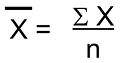# Pandas: Filling missing values by mean in each group

Given a Pandas DataFrame, we have to fill missing values by mean in each group.
Submitted by Pranit Sharma, on July 04, 2022

Pandas is a special tool that allows us to perform complex manipulations of data effectively and efficiently. Inside pandas, we mostly deal with a dataset in the form of DataFrame. DataFrames are 2-dimensional data structures in pandas. DataFrames consist of rows, columns, and data.

The most fascinating key point about pandas is that it contains numerous amounts of function to calculate almost everything mathematically and logically. With the help of pandas, we can calculate the mean of any column in a DataFrame, the column values should be integer or float values and not string.

## pandas.DataFrame[col].Mean()

Mean is nothing but an average value of a series of a number. Mathematically, the mean can be calculated as:Here, x̄ is the mean, x is the summation of all the values and n is the total number of values/elements.

Suppose we have a series of numbers from 1 to 10, then the average of this series will be:

```∑x = 1+2+3+4+5+6+7+8+9+10
∑x = 55
n = 10
x̄ = 55/10
x̄ = 5.5
```

But in pandas, we use pandas.DataFrame['col'].mean() directly to calculate the average value of a column.

To fill missing values by mean in each group, we will first groupby the same values and then fill the NaN values with their mean.

To work with pandas, we need to import pandas package first, below is the syntax:

```import pandas as pd
```

Let us understand with the help of an example,

## Python code to fill missing values by mean in each group

```# Importing pandas package
import pandas as pd

# Importing numpy package
import numpy as np

# Creating a dictionary
d = {
'Name':['Ram','Shyam','Bablu','Shyam','Bablu','Ram'],
'Marks':[20,np.NaN,18,19,21,np.NaN]
}

# Creating a DataFrame
df = pd.DataFrame(d)

# Display original DataFrame
print("Original DataFrame:\n",df,"\n")

# Filling nan values with mean
df["Marks"] = df.groupby('Name').transform(lambda x: x.fillna(x.mean()))

# Display result
print("Modified Dataframe:\n",df)
```

Output:

```Original DataFrame:
Name  Marks
0    Ram   20.0
1  Shyam    NaN
2  Bablu   18.0
3  Shyam   19.0
4  Bablu   21.0
5    Ram    NaN

Modified Dataframe:
Name  Marks
0    Ram   20.0
1  Shyam   19.0
2  Bablu   18.0
3  Shyam   19.0
4  Bablu   21.0
5    Ram   20.0
```

Top MCQs